### Contest Math I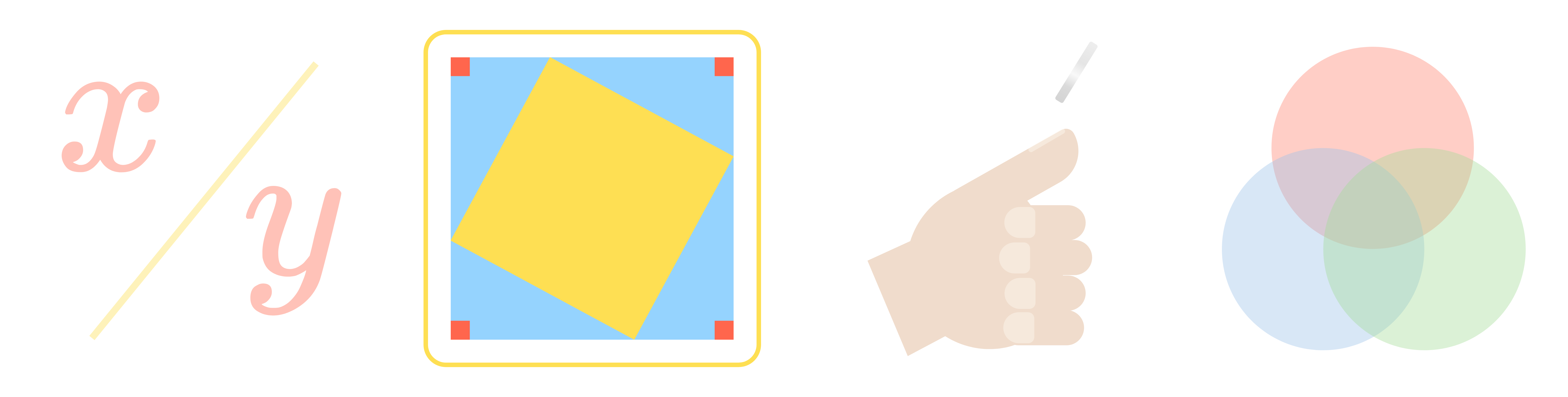When you're solving a challenging puzzle, strategy matters! The most obvious way to start solving the problem or the first method that you think of is not always the best way.

In geometry problems, figures can often be "transformed" by rotating them, stretching them, combining them, or breaking them apart. While these transformations are not always necessary to reach an answer, they can often lead to a more efficient solution than the more obvious approach.

As you work through these geometry problems, look for ways to transform the figures to find these solutions.

# Geometry

Which triangle has an area of $1?$# Geometry

$10$ unit blocks are painted red, then assembled into this staircase as shown. The staircase is then painted blue on every visible surface (the top, front, back, and both of the sides, but not the bottom.)

When the staircase is disassembled, what total surface area will still be painted red, among all $10$ blocks?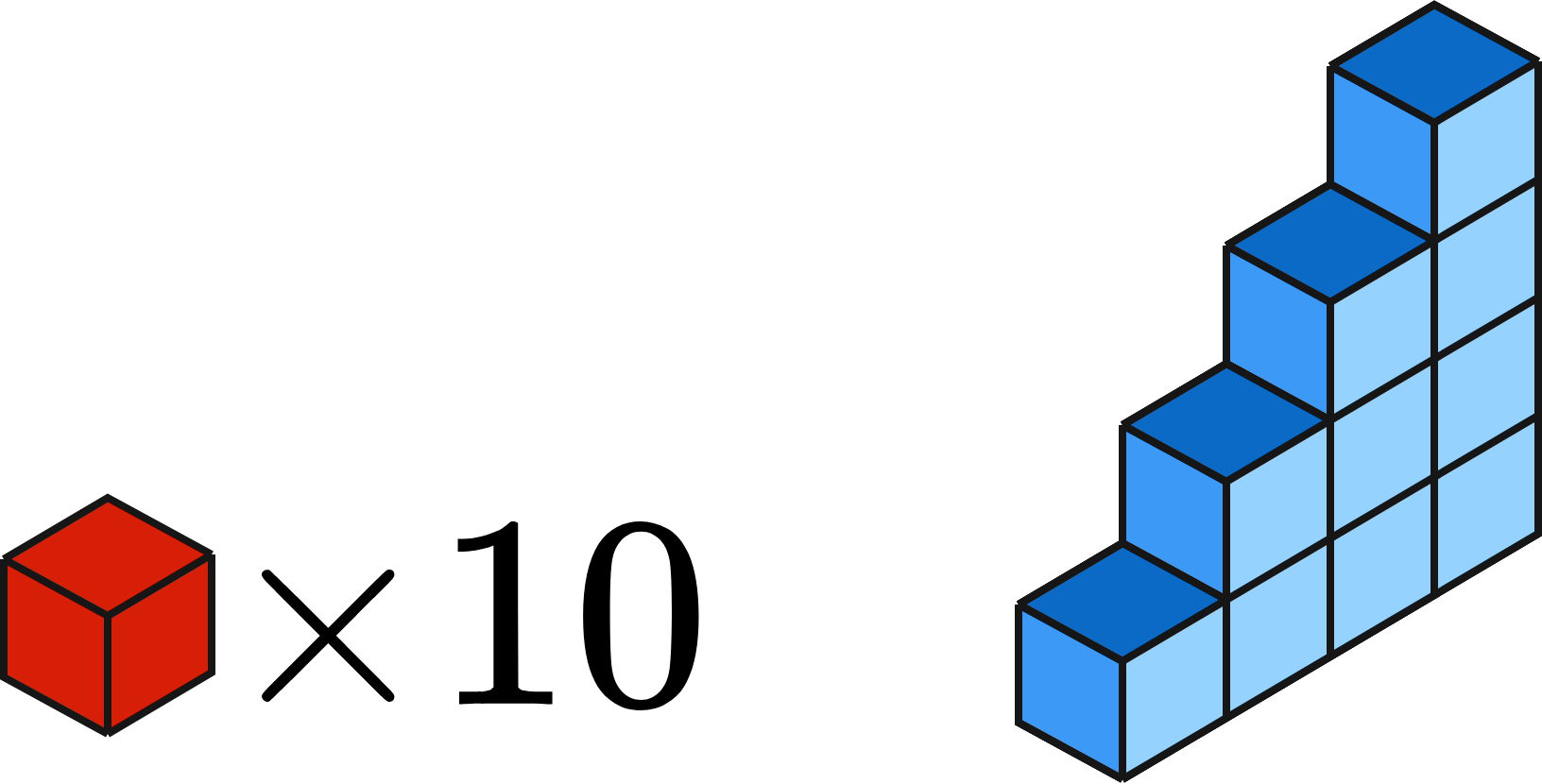# Geometry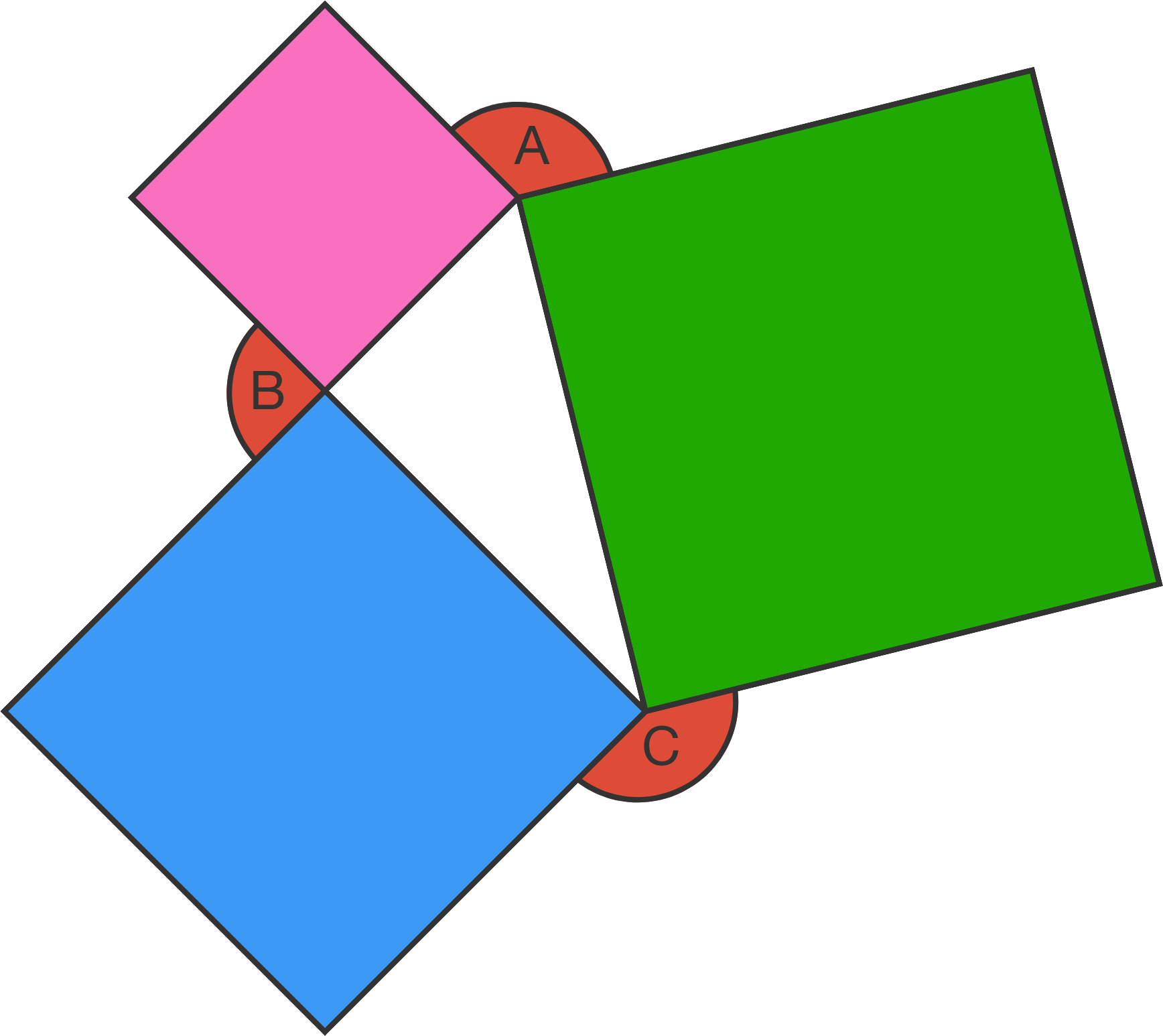Three squares are joined together as shown in the figure to create a triangle.

What is the sum of the measures of angles $A,$ $B,$ and $C?$

# Geometry

A lattice triangle is a triangle whose vertices coincide with points on a regular grid.

Which of the lattice triangles below has the greatest area?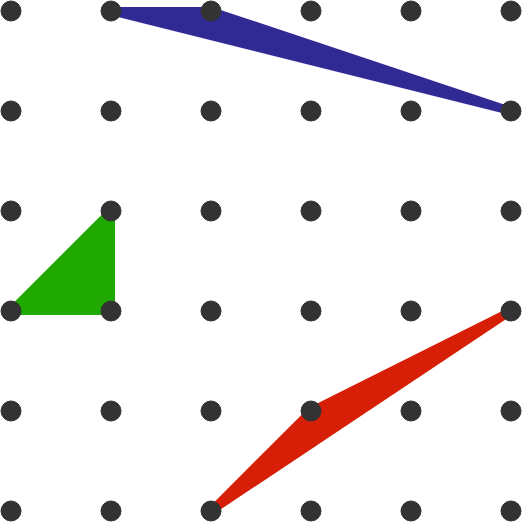# Geometry

The figure shows a square inscribed in a circle inscribed in a square. If the side length of the largest square is $10,$ what is the area of the red region?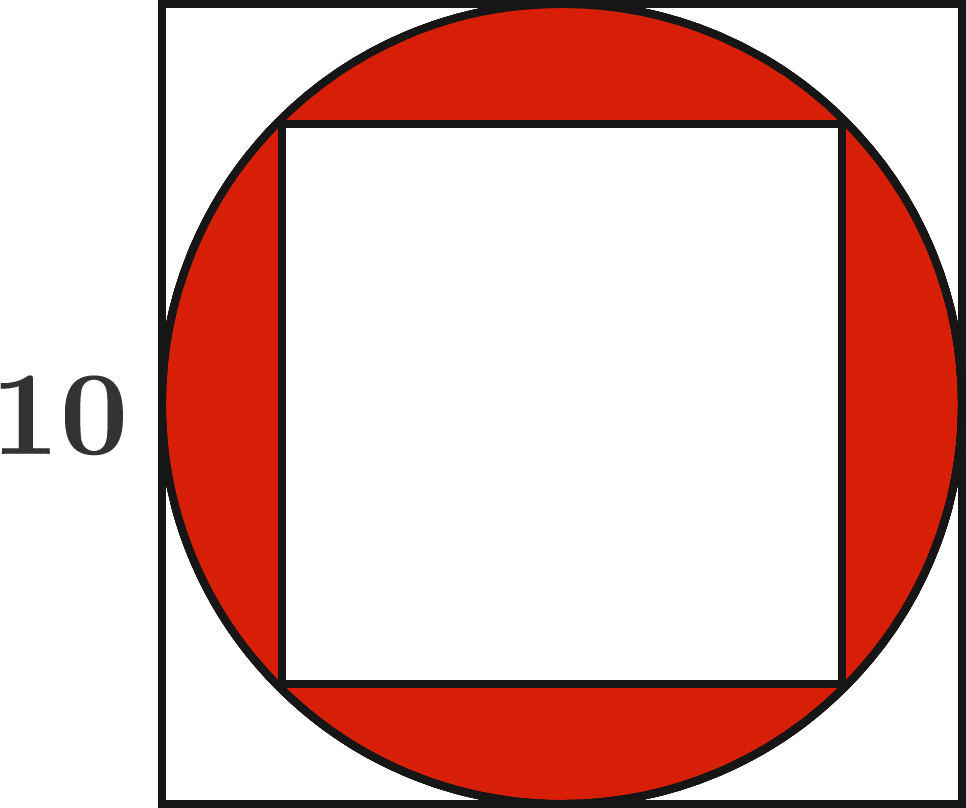# Geometry

The geometry questions in math competitions frequently focus on finding missing measurements, comparing similar figures, and combining simple shapes to create more complicated diagrams. We have units on each of these categories in this course, and you don't need to work through all of the units in this course in order, you can jump directly to whichever one you want to practice.

Our unit on Measures will teach you techniques and equations that will help you calculate unknown lengths, areas, and volumes of 2D and 3D shapes. To learn more, you can either explore what's in this unit or read through the Geometry: Measures Syllabus.

Our unit on Similarity will dive into the relationships between similar figures, and show how to use them to calculate unknown lengths and areas. To learn more, you can either explore what's in this unit or read through the Geometry: Similarity Syllabus.

Our unit on Composites will help you develop strategies for measuring complex shapes by breaking them down into simpler ones. To learn more, you can either explore what's in this unit or read through the Geometry: Composites Syllabus.

×